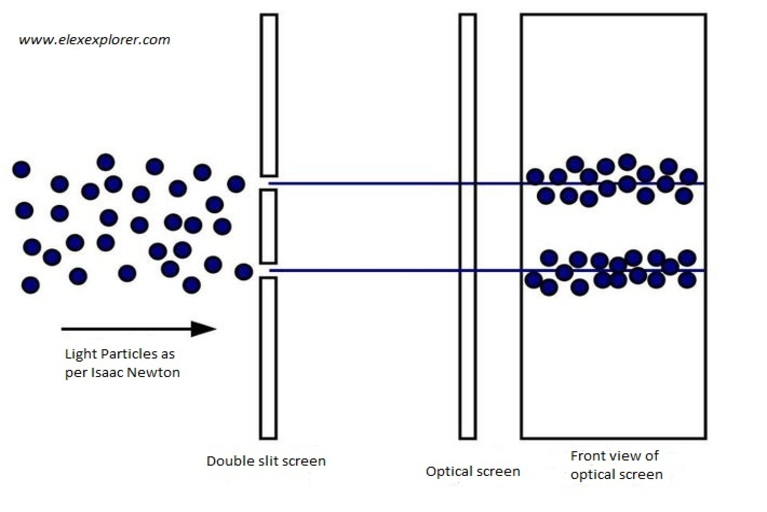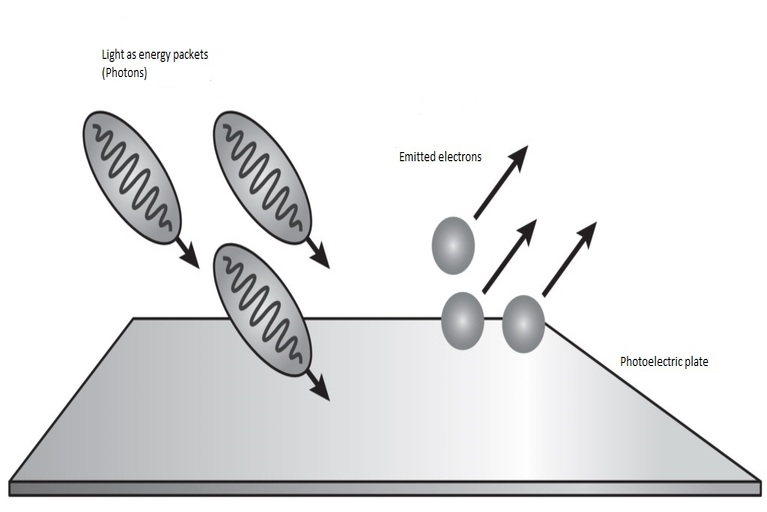In our childhood, we have seen many superheroes that were moving faster than bullets. We could even picture them multiple times with amazing expressions. If our superhero traveled at half of the bullet’s speed, the rate at which the bullet moved away from him would halve. But now there is one more thing that is moving with a faster speed than bullets and that is “Light”. Is there anything that can move faster than light? Let’s find out.

## Science Behind Light:

In 1700 Newton published his treatise Opticks, this was 17 years after his great work Principia. Opticks was written in English and became very popular. In his research, Newton proposed that light consists of little particles. It means that light is moving from one point to another in the form of small particles. As per this theory if a horizontal beam of light near the earth is undergoing projectile motion then it will form a parabolic path. In this parabolic path, the straight line we observe is due to the fact that the speed of the particles is very high. In one microsecond, light travels 300 meters.Light as particle as per NewtonLight as photon as per Einstein

Then a German physicist and mathematician the famous Albert Einstein came along and changed the image of light behavior. Einstein found that light is made of small energy packets which are known as photons. In his publication ‘Theory of special relativity’ Einstein provides a formula E = mc2. Here E is energy, m is mass and c is the speed of light.

According to the equation E = mc2, mass and energy are the same physical element and they can be interchangeable. It means that an increment in energy also increases its mass. In simple words, the faster an object moves, the greater it’s mass. This change is noticeable only when an object moves very quickly. Suppose, if an object moves at 10% of the speed of light then its mass only will increase by 0.5% whereas if it moves at 90% of the speed of light then its mass will double.

If an object moves at the speed of light then its mass becomes infinite, and it also needs infinite energy to move. For this reason, no normal object can move with a speed equal to or greater than the speed of light.

## If We Got Speed of Light:

As per Einstein ‘Time’ is also relative. If we got the speed of light then we would experience a very fascinating effect that is ‘Time dilation’. The time dilation effect shows how time runs more slowly for objects those are moving very rapidly. It means if you travel in a spaceship that is moving with the speed of light then you will become a time traveler.

## What is Time Dilation?

The word time dilation refers to the seemingly odd fact that time passes at different rates for different observers, depending on their relative motion or positions in a gravitational field. It means time is relative.

How can time slow down at really fast speeds? Well, to answer this question, we need to consider how we measure time. Time is measured by the observation of repeating events. For example, All clocks record time with some repeating event, such as a pendulum or crystal that moves back and forth.

As of now, human beings are still trying to get the speed of light. Hopefully, it will happen one day.

Thanks for reading. See you soon with another exploration!